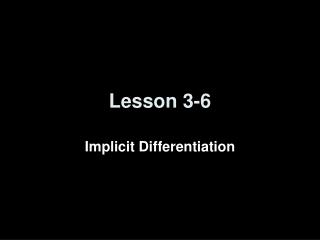# Lesson 3-6 - PowerPoint PPT PresentationDownload PresentationLesson 3-6

Lesson 3-6
Download Presentation## Lesson 3-6

- - - - - - - - - - - - - - - - - - - - - - - - - - - E N D - - - - - - - - - - - - - - - - - - - - - - - - - - -
##### Presentation Transcript

1. Lesson 3-6 Implicit Differentiation

2. Objectives • Use implicit differentiation to solve for dy/dx in given equations • Use inverse trig rules to find the derivatives of inverse trig functions

3. Vocabulary • Implicit Differentiation – differentiating both sides of an equation with respect to one variable and then solving for the other variable “prime” (derivative with respect to the first variable) • Orthogonal – curves are orthogonal if their tangent lines are perpendicular at each point of intersection • Orthogonal trajectories – are families of curves that are orthogonal to every curve in the other family (lots of applications in physics (example: lines of force and lines of constant potential in electricity)

4. Guidelines for Implicit Differentiation • Differentiate both sides of the equation with respect to x. • Collect all terms involving dy/dx on one side of the equation and move all other terms to the other side. • Factor dy/dx out of the terms on the one side. • Solve for dy/dx by dividing both sides of the equation by the factored term.

5. Example 1 Find the derivatives of the following: • x = y² - xy + x³ • y = (e4x²) (cos 6y) 1 = 2y (dy/dx) – y – x(dy/dx) + 3x² 1 + y – 3x² = (2y – x) (dy/dx) dy/dx = (1 + y – 3x²) / (2y – x) (dy/dx) = 8x (e4x²) (cos 6y) + (e4x²) (-6sin 6y)(dy/dx) -8x (e4x²) (cos 6y) = ((e4x²) (-6sin 6y) – 1))(dy/dx) -8x (e4x²) (cos 6y) / ((e4x²) (-6sin 6y) – 1)) = dy/dx

6. Example 2 Find the derivatives of the following: • 0 = 360x² + 103xy – 16xy² • 6 = (tan y²) (sin 4x³) 0 = 720x + 103x(dy/dx) + 103y – 32xy(dy/dx) – 16y² 16y² – 720x – 103y = (dy/dx)(103x – 32xy) dy/dx = (16y² – 720x – 103y) / (103x – 32xy) 0 = (sec² y²)(2y)(dy/dx)(sin 4x³) + (tan y²)(cos 4x³)(12x²) (tan y²)(cos 4x³)(-12x²) = (sec² y²)(2y)(sin 4x³)(dy/dx) dy/dx = (-6x²) (tan y²)(cos 4x³) / (y)(sec² y²)(sin 4x³)

7. Example 3 Find the derivatives of the following: • y = xy + x²y³ • 25 = (tan 2x) (3y³ - 4y² + 7y - 9) (dy/dx) = x(dy/dx) + y + 2xy³ + 3x²y²(dy/dx) -2xy³ - y – 3x²y² = (x + 3x²y² - 1) (dy/dx) dy/dx = (-2xy³ - y – 3x²y² ) / (x + 3x²y² - 1) 0 = (sec 2x)(2)(3y³ - 4y² + 7y – 9) + (9y² - 8y + 7)(dy/dx)(tan 2x) -(sec 2x)(2)(3y³ - 4y² + 7y – 9) = (9y² - 8y + 7)(tan 2x)(dy/dx) dy/dx = (-(sec 2x)(2)(3y³ - 4y² + 7y – 9) ) / ((9y² - 8y + 7)(tan 2x))

8. Summary & Homework • Summary: • Use implicit differentiation when equation can’t be solved for y = f(x) • Derivatives of inverse trig functions do not involve trig functions • Homework: • pg 233-235: 1, 6, 7, 11, 17, 25, 41, 47